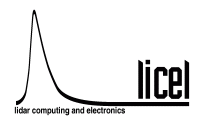Licel Raw Data FormatThe header lines (ASCII format) have a length of at least 80 characters, where the two last characters are <CRLF>. The header lines are followed by the data in binary format. The first header line contains the filename,

• which begins with one or two letters
• two digits showing the years in the century
• the month (hexadecimal, one digit)
• the day (decimal, two digits)
• the hour (decimal, 24 hours per day, two digits)
• a period (.)
• the minute (decimal, two digits)
• the seconds (decimal, two digits)
• two or three decimal places of the seconds (decimal, two or three digits).

• measurement site (8 characters)
• measurement start time (dd/mm/YYYY HH:MM:SS)
• measurement finish (dd/mm/YYYY HH:MM:SS)
• the altitude above sea level (four digits (meter))
• the longitude (11 digits (including - sign), six decimal places)
• the latitude (11 digits (including - sign), six decimal places)
• the zenith angle (four digits in degrees), one decimal place)
• the azimuth angle (four digits in degrees), one decimal place)
• a custom information field enclosed by quotation marks ("") [optional]

• the number of laser shots of the type 1 laser (integer 7 digits)
• the repetition rate of the Type 1 laser (integer 4 digits)
• the number of laser shots of the type 2 laser (integer 7 digits)
• the repetition rate of the Type 2 laser (integer 4 digits)
• the number of the following data sets (integer 2 digits) are given
• the number of laser shots of the type 3 laser (integer 7 digits)
• the repetition rate of the Type 3 laser (integer 4 digits)
• 0000000 0000 (reserved)
• the controller timestamp corresponding to the data [optional]

The header lines specific to the data sets contain:

• data set active (1|0). Non-active data sets will not be written.
• data set type. 0 Analog, 1 Photon Counting, 2 Analog squared, 3 Photon Counting squared
• laser (1|2|3)
• number of data points (5 digits)
• the laser polarization 0 (none, vertical, horizontal, right circular, left circular) 0|1|2|3|4
• High voltage of the PM (V) (four digits in Volt)
• bin width (in meters four digits including decimal separator (.) and decimal places)
• wavelength (nm) in nm, five digits followed by a period (.)
• polarization status (none, parallel, crossed, right circular, left circular) 0|p|s|r|l
• 0 0 (backward compatibility)
• bin shift, whole-number (primary bins, integer rounded down, 2 digits, 00 if not supported or zero)
• decimal places of the bin shift (3 digits, 000 if not supported or zero)
• the number of bits of the ADC (2 digits)
• the number of shots (6 digits)
• discriminator level or data range of the ADC (one digit period 4/3 digits)
• device identificator
• a custom information field enclosed by quotation marks ("") [optional]. If a transient recorder channel is assigned to a detector<detectorID: detector description\textgreater> will be part of the custom info field which is always present in that case.
•  BT analog dataset BC photon counting PD powermeter (photodiode) PM powermeter (powermeter) S2A s sqrt(N(N-1)) (analog, s sample standard deviation, N shots) S2P s sqrt(N(N-1)) (photon counting, s sample standard deviation, N shots)
(The number is the address of the transient recorder or power meter detector as a hexadecimal number)

The header lines are followed by the 32 bit data sets. Each data set is introduced by a <CRLF>. After the last data set another <CRLF> follows.

The data sets can be converted to quantities with physical units by using the number of shots and additionally the number of bits and the data range in case of the analog signal. This procedure is executed e.g. in Advanced Viewer.exe

Back to index